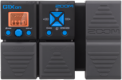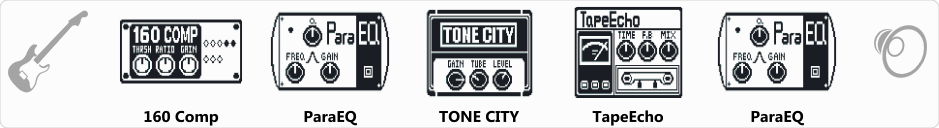# Jazz direct

Discussion in 'Zoom G1on/G1Xon' started by Kovács László, Mar 13, 2021.

1. Jazz directDevice: Zoom G1Xon
Firmware: 1.21

Name on device: IBANEZ4SOL
Optimized for: Phones/Speaker

Effects chain:Jazzy sound , enough good with cheep pickups.

Effect: "160 Comp" (Dynamics / Filter), active - "yes"
"THRSH" = -38
"Ratio" = 3.6
"Gain" = 4
"Knee" = Hard
"Level" = 120

Effect: "ParaEQ" (Dynamics / Filter), active - "yes"
"Freq1" = 250Hz
"Q1" = 1
"Gain1" = 7
"Freq2" = 2.5kHz
"Q2" = 1
"Gain2" = 11
"Level" = 141

Effect: "TONE CITY" (Amp simulators), active - "yes"
"Gain" = 35
"Tube" = 30
"Level" = 148
"Trebl" = 54
"Middl" = 50
"Bass" = 46
"Prese" = 10
"CAB" = ALIEN 4x12
"OUT" = LINE

Effect: "TapeEcho" (Delay / Reverb), active - "yes"
"Time" = 435
"F.B" = 45
"Mix" = 25
"HiDMP" = 10
"Level" = 100
"Tail" = On

Effect: "ParaEQ" (Dynamics / Filter), active - "yes"
"Freq1" = 250Hz
"Q1" = 0.5
"Gain1" = 5
"Freq2" = 2.5kHz
"Q2" = 1
"Gain2" = 5
"Level" = 100

Patch Volume: 100

Note: This is a patch file, you will need to download and install the ToneLib-Zoom software to use the patch.

File size:
440 bytes
Views:
228